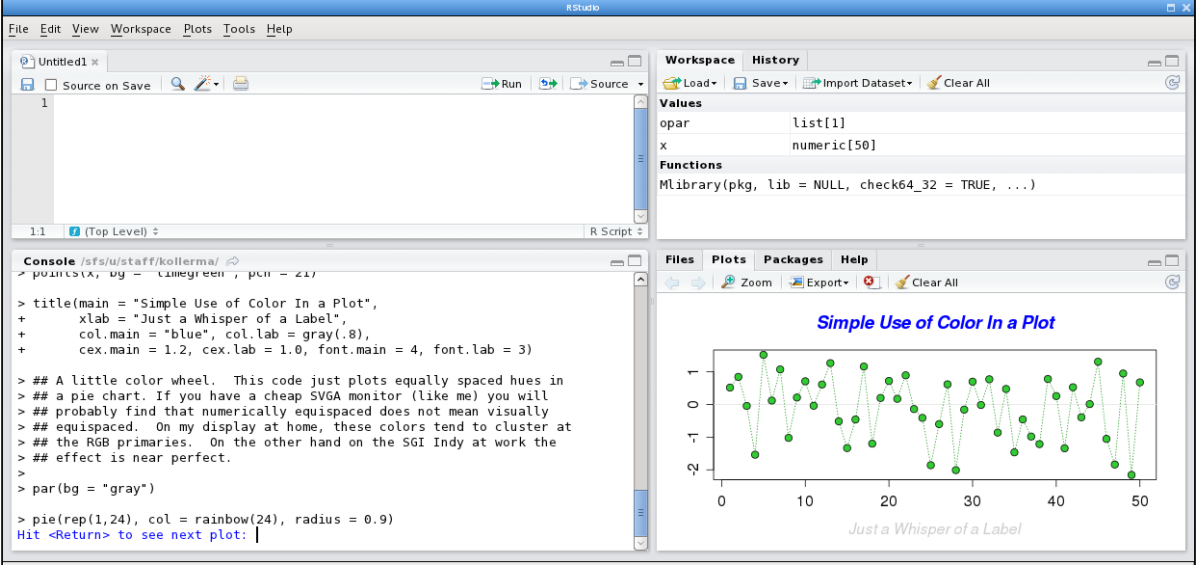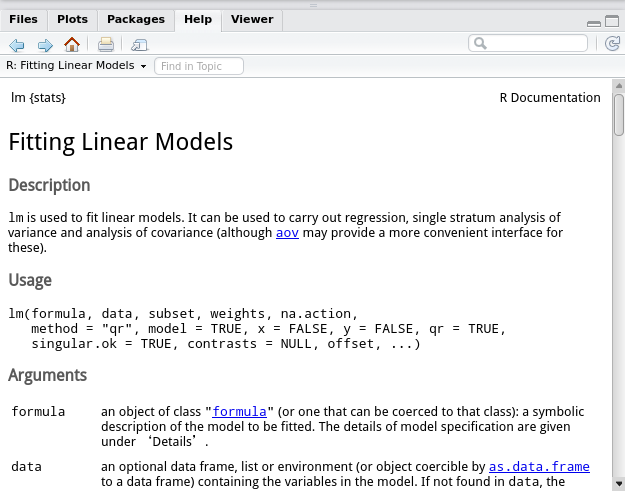# R语言从入门到放弃 (1). 基础

## terminology

• `predictor variable`: 就是机器学习里面说的feature (Xi)
• `design points`: 是机器学习里的训练数据(X1...Xn)
• `response variable`: 要预测的变量(y)

## Rstudio

• 默认有四个窗口: 编辑器, 变量, 命令行, 画图/帮助.## 快捷键

• `alt+-`: 用于输入赋值符号`<-`
• (编辑器窗口中) `ctrl+enter`: (在console里)执行光标所在的行, 等同于按钮"run"
• (r markdown编辑器窗口中) `ctrl+alt+I`: 插入一个R代码的chunk
• (r markdown编辑器窗口中) `ctrl+shift+enter`: 运行当前code chunk
• (选中一个函数名) `F1`: 在右下角帮助窗口里显示相关文档.

## R 文档

```demo(graphs)
example("plot")
```Values里的每一项是这个函数的返回值(object)的内容, 比如要获得一个lm object的参数, 只需要: `lm_obj\$coefficients`

## 常用函数

• `c(...)`

combine values into vector/list. 注意的是它会自动把list/vector参数展开:

```> c(1,2,3)
 1 2 3
> c(c(1,2),3)
 1 2 3
```

`l <- c(l, new_element)`

• `numeric(l)`

```> numeric(5)
 0 0 0 0 0
```

• `matrix(v, nrow=nr, ncol=nc)`

• `seq(from, to, by)`

```> seq(1,10, 2)
 1 3 5 7 9
> 1:5
 1 2 3 4 5
```
• for循环: `for(i in 1:n){...`

• `rep`

```> rep(1, 10)
 1 1 1 1 1 1 1 1 1 1
> rep(c(1,2), 10)
 1 2 1 2 1 2 1 2 1 2 1 2 1 2 1 2 1 2 1 2
> rep(c(1,2), each=10)
 1 1 1 1 1 1 1 1 1 1 2 2 2 2 2 2 2 2 2 2
```
• `length(lst)`

• `dim(mat)`

• `cbind/rbind`

```> cbind(c(1,2),c(3,4))
[,1] [,2]
[1,]    1    3
[2,]    2    4
```
• `str`/`summary`

• `cat`/`print`

• `which`/`which.max`/`which.min`

which.max/min类似于argmax/min

```> which(2>c(1,2,1))
 1 3
> which.max(c(1,2,1))
 2
> which.min(c(1,2,1))
 1
```
• `paste`

```> paste("a", 1:5, sep="-")
 "a-1" "a-2" "a-3" "a-4" "a-5"
```
• operators: with `%`